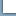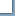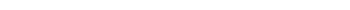Fibonacci NumbersThe Fibonacci Series is a sequence of numbers first created by Leonardo Fibonacci in 1202. First number is one and then each number of the series is the sum of the two numbers preceding it.1 Trading Plan2 Money Management 3 Technical Tools 4 Trading Types 5 Trading Psychology 6 Stop Loss 7 Trading Goals 8 Market Profile 9 Volatility Index (VIX) 10 FibonacciINVESTING1 Investment Goals2 Risk Tolerance 3 Investor Profile 4 Asset Allocation 5 Portfolio Management 6 Portfolio Compensation 7 Portfolio Benchmark 8 Portfolio ModelFibonacci << Prev - Nex >>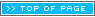Read More...Fed Fund RateBank Prime Rate30-Year Fixed RateLiborMBA Mortgage ApplicationsGlobal ViewRegisterFIBONACCI

Fibonacci Series

0, 1, 1, 2, 3, 5, 8, 13, 21, 34, 55, 89, 144, 233, 377, 610, 987, 1597, 2584, 4181, 6765, 10946...

Golden Ratio

The Golden Rectangle is proposed to be the most aesthetically pleasing of all possible rectangles. For this reason, it and the Golden Ratio have been used extensively in art and architecture for thousands of years. The most prominent and well known uses of the Golden Rectangle in art were created by the great Italian artist, inventor, and mathematician, Leonardo da Vinci.

Fibonacci Ratios

The last three sections have done much to explain Golden geometry, but haven't explained how they relate to the topic of the Fibonacci series. Actually, the Golden Ratio and the Fibonacci numbers are two concepts that have a lot to do with each other.
The most important appearance of the Golden Ratio in Fibonacci mathematics is the Fibonacci ratio sequence. The Fibonacci ratio sequence is the series of numbers you get when you divide each Fibonacci number with the one that precedes it. Let's look at a few terms of this sequence.

f(1) = 1
f(2) = 1
f(3) = 2
f(4) = 3
f(5) = 5
f(6) = 8
f(7) = 13
f(8) = 21

In order to create the first few terms of the sequence, we divide each Fibonacci number by the one before it. Since there is no Fibonacci number before f(1), we start with f(2). Dividing f(2) by f(1) gives a value of 1, so 1 is the first number of the Fibonacci ratio sequence, or r(1). Then we divide f(3) by f(2) to get the value of r(2), 2. Continuing in this fashion, we get:

r(1) = 1 / 1 = 1
r(2) = 2 / 1 = 2
r(3) = 3 / 2 = 1.5
r(4) = 5 / 3 = 1.67
r(5) = 8 / 5 = 1.6
r(6) = 13 / 8 = 1.625
r(7) = 21 / 13 = 1.615

Fibonacci numbers appear in this infinite sequence: 0, 1, 1, 2, 3, 5, 8, 13, 21, 34 , where each number is the sum of the previous two. Divide one Fibonacci number by its predecessor, and the quotients will cluster around 1.618 -- the golden mean, or so-called divine proportion. Technical analysts, as opposed to those who rely on fundamentals like earnings and economic growth, say that both can be used to predict a market’s direction.

Markets are governed by waves that crest and fall based on a sequence of numbers called the Fibonacci sequence and the closely related golden mean, or golden ratio.

Gann Angles

W D Gann was an innovator in technical trading systems and made fortune of over 50 million dollars Gann died in 1955, but his methods live on and traders around the world use them for big profits.

Gann angles are a tool used by many savvy traders Quite simply Gann angles allow you to pinpoint your entry and exit levels for great profit potential. Let's look at the effectiveness of Gann angles.

Gann based his investment strategy on the fact that by studying the Past, We Can Predict the Future. He assumed the following:

1. Price, time, and range are the only three factors relevant to market movement.

2. Markets are cyclical in nature.

3. The markets are geometric in their design and in function. Gann knew that market movements were a reflection of human nature which is constant over time, and this shows up in repetitive price patterns that can be seen with the right tolls and traded for profit.

Gann identified nine significant angles, with the 1 x 1 being the most important:

1 x 8 = 82.5 degrees
1 x 4 = 75 degrees
1 x 3 = 71.25 degrees
1 x 2 = 63.75 degrees
1 x 1 = 45 degrees
2 x 1 = 26.25 degrees
3 x 1 = 18.75 degrees
4 x 1 = 15 degrees
8 x 1 = 7.5 degreesGLOBAL VISION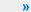Reports ViewETFsMarketsECONOMICSStocksFuturesFibonacciCALENDARResearchWeeklyS&P 500PERFORMANCETradingInvestingSlidesLOG-INRegisterPricingSitemapABOUTContactHelpFAQsYou can follow us at: